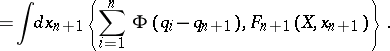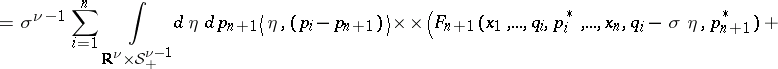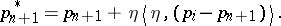# BBGKY hierarchy

(diff) ← Older revision | Latest revision (diff) | Newer revision → (diff)

Bogolyubov–Born–Green–Kirkwood–Yvon hierarchy

A chain of an infinite number of integro-differential evolution equations for the sequence of distribution (correlation) functions describing all possible states of a many-particle system. For systems of finitely many particles the BBGKY hierarchy is equivalent to the Liouville equation in the classical case and to the von Neumann equation in the quantum case.

Let $F ( t ) = ( F _ { 1 } ( t , x _ { 1 } ) , \ldots , F _ { n } ( t , x _ { 1 } , \ldots , x _ { n } ) , \ldots )$ be a sequence of probability distribution functions $F _ { n } ( t )$ defined on the phase space of a system of $n$ particles $x _ { i } \equiv ( q _ { i } , p _ { i } ) \in \mathbf{R} ^ { \nu } \times \mathbf{R} ^ { \nu }$, $\nu \geq 1$ (cf. also Distribution function). The BBGKY hierarchy for classical systems reads as follows:

\begin{equation*} \frac { d } { d t } F ( t ) = - \mathcal{L} F ( t ) + [ \mathcal{L} , A ] F ( t ), \end{equation*}

where the Liouville operator $\mathcal{L}$ is defined by the Poisson brackets ($X \equiv ( x _ { 1 } , \dots , x _ { n } )$):

\begin{equation*} ( \mathcal{L} F ) _ { n } ( X ) = \{ H _ { n } , F _ { n } ( X ) \} \end{equation*}

with Hamiltonian $H _ { n } = \sum _ { i = 1 } ^ { n } p _ { i } ^ { 2 } / 2 + \sum _ { 1 = i < j } ^ { n } \Phi ( q _ { i } - q _ { j } )$ and the symbol $[ \cdot , \cdot ]$ denotes the commutator between the operator $\mathcal{L}$ and the operator

\begin{equation*} ( \mathcal{A} F ) _ { n } ( X ) = \int d x _ { n + 1} F _ { n + 1} ( X , x _ { n + 1} ). \end{equation*}

The main examples of the BBGKY hierarchy are discussed below [a1].

1) For a smooth potential of interaction $\Phi$,

\begin{equation*} ( [ {\cal L , A} ] F ) _ { n } ( X ) = \end{equation*}2) For a system with singular potential of interaction

\begin{equation*} \Phi ( q ) = \left\{ \begin{array} { l l } { + \infty } & { \text { if } | q | \leq \sigma , } \\ { 0 } & { \text { if } | q | > \sigma , } \end{array} \right. \end{equation*}

like a hard-sphere system of diameter $\sigma$, for dimension $\nu \geq 2$ at $t > 0$ one has

\begin{equation*} ( [ {\cal L , A} ] F ) _ { n } ( X ) = \end{equation*}\begin{equation*} - F _ { n + 1 } ( X , q _ { i } + \sigma \eta , p _ { n + 1 } ) ), \end{equation*}

where $\langle \, .\, ,\, . \, \rangle$ is the inner product,

\begin{equation*} \mathcal{S} _ { + } ^ { \nu - 1 } = \left\{ \eta \in \mathbf{R} ^ { \nu } : | \eta | = 1 , \langle \eta , ( p _ { i } - p _ { n + 1 } ) \rangle > 0 \right\} \end{equation*}

and

\begin{equation*} p _ { i } ^ { * } = p _ { i } - \eta \langle \eta , ( p _ { i } - p _ { n + 1 } ) \rangle, \end{equation*}3) The dual BBGKY hierarchy

\begin{equation*} \frac { d } { d t } G ( t ) = \mathcal{L} G ( t ) + [ \mathcal{L} , \mathcal{A} ^ { * } ] G ( t ), \end{equation*}

where $\mathcal{A} ^ { * }$ is the adjoint operator to $\mathcal{A}$:

\begin{equation*} ( \mathcal{A} ^ { * } f ) _ { n } ( X ) = \sum _ { i = 1 } ^ { n } f _ { n - 1 } ( x _ { 1 } , \dots , x _ { i - 1} , x _ { i + 1} , \dots , x _ { n } ). \end{equation*}

Such a hierarchy describes the evolution of observables $G ( t )$ of a system of particle.

There are two different approaches to constructing the solutions of the hierarchy: either by iteration or by a functional (cluster expansion) series; see [a1]. The functional representation of the solution for the Cauchy problem depends on symmetry properties of the Hamiltonian and on initial distribution functions

\begin{equation*} F ( 0 ) = ( F _ { 1 } ( 0 , x _ { 1 } ) , \ldots , F _ { n } ( 0 , x _ { 1 } , \ldots , x _ { n } ) , \ldots ). \end{equation*}

In the symmetric case it has the form

\begin{equation*} F _ { s } ( t , x _ { 1 } , \ldots , x _ { s } ) = \end{equation*}

\begin{equation*} = \sum _ { n = 0 } ^ { \infty } \int d x _ { s + 1} \cdots d x _ { s + n} U ^ { ( n )_t } F _ { s + n} ( 0 , x _ { 1 } , \dots , x _ { s + n} ), \end{equation*}

where

\begin{equation*} U ^ { ( n )_ t} = \sum _ { k = 0 } ^ { n } \frac { ( - 1 ) ^ { k } } { k ! ( n - k ) ! } S ^ { s + n - k } ( - t , x _ { 1 } , \dots , x _ { s + n - k} ) \end{equation*}

and the evolution operator $S ^ { n } ( - t , x _ { 1 } , \dots , x _ { n } )$,

\begin{equation*} S ^ { n } ( - t , x _ { 1 } , \dots , x _ { n } ) F _ { n } ( x _ { 1 } , \dots , x _ { n } ) = \end{equation*}

\begin{equation*} = F _ { n } ( X _ { 1 } ( - t , x _ { 1 } , \ldots , x _ { n } ) , \ldots , X _ { n } ( - t , x _ { 1 } , \ldots , x _ { n } ) ), \end{equation*}

shifts the arguments of the functions along the solutions of the Hamilton equations (the phase trajectories) $X _ { i } ( - t , x _ { 1 } , \ldots , x _ { n } )$, $i = 1 , \dots , n$, of a system of $n$ particles with initial data $X _ { i } ( 0 , x _ { i } ) = x _ { i }$. The evolution operators $S ^ { n } ( t )$ form a group of operators generated by the Liouville operator ${\cal L} _ { n }$ defined above. In certain cases this representation of the solution (a cluster expansion) can be treated as the solution $F ( t ) = U ( t ) F ( 0 )$ determined by a group of evolution operators $U ( t )$. In terms of the operators defined above, the group $U ( t )$ has the form

\begin{equation*} U ( t ) = e ^ { \mathcal{A} } S ( - t ) e ^ { - \mathcal{A} }. \end{equation*}

There exist various methods to give a meaning to the formal solution of the Cauchy problem to the BBGKY hierarchy in certain function spaces. For example, the semi-group method (in spaces of sequences of summable functions and, for quantum systems, in the space of sequences of kernel operators), the interaction region method (for constructing an a priori estimate of a solution), the thermodynamic limit method (for the time-continuation of the local solution in the space of sequences of bounded functions), and non-standard analysis methods.

To date (1998), existence and uniqueness results for the BBGKY hierarchy have been proved:

a) in the space $L^1$ of sequences of summable functions (or kernel operators, in the quantum case): time-global solutions for general classes of an interaction potential;

b) in the space $L^{\infty}$ of sequences of functions bounded with respect to the configuration variables and decreasing with respect to the momentum variables: time-global solutions for the BBGKY hierarchy of a hard-sphere system for arbitrary initial data in the one-dimensional case and with initial data close to the equilibrium state in the many-dimensional case; also, time-global solutions for one-dimensional systems of particles interacting by the short-range potential with a hard-core and with initial data close to equilibrium.

The BBGKY hierarchy allows one to describe both equilibrium and non-equilibrium states of many-particle systems from a common point of view. Non-equilibrium states are characterized by the solutions of the initial value problem for this hierarchy and, correspondingly, equilibrium states are characterized by the solutions of the steady BBGKY hierarchy, see [a1], [a3], [a2].

In so-called large-scale limits, asymptotics of solutions to the initial value problem of the BBGKY hierarchy are governed by non-linear kinetic equations (cf. also Kinetic equation) or by hydrodynamic equations depending on initial data. For example, in the Boltzmann–Grad limit the asymptotics of solutions of the BBGKY hierarchy is described by the Boltzmann hierarchy and, as a consequence, for factorized initial data the equation determining the evolution of an initial state is the Boltzmann equation.

General references for this area are [a1], [a4], [a3], [a2], [a5].

How to Cite This Entry:
BBGKY hierarchy. Encyclopedia of Mathematics. URL: http://encyclopediaofmath.org/index.php?title=BBGKY_hierarchy&oldid=50793
This article was adapted from an original article by V.I. Gerasimenko (originator), which appeared in Encyclopedia of Mathematics - ISBN 1402006098. See original article